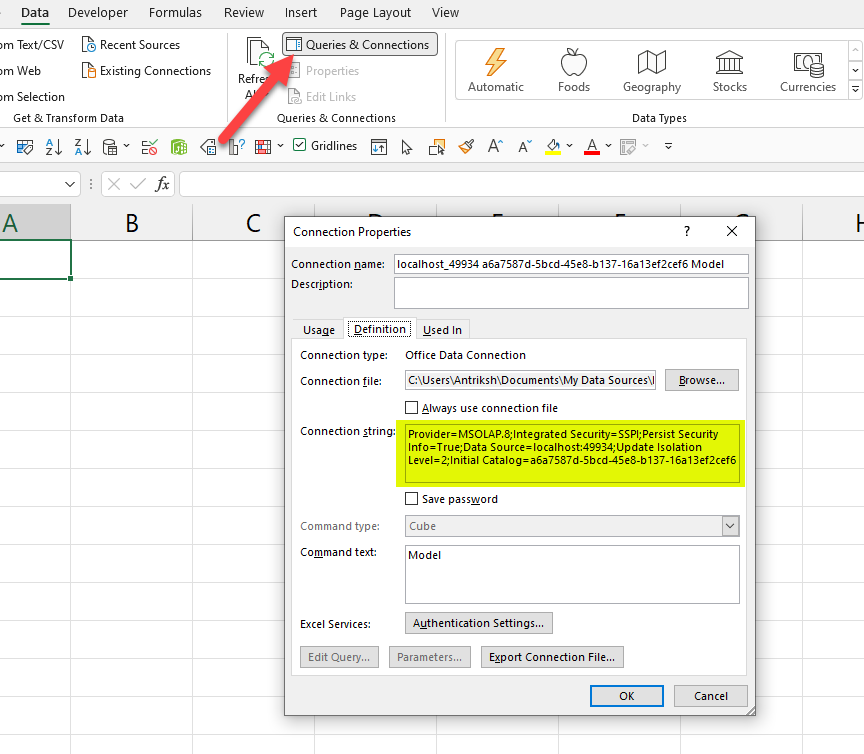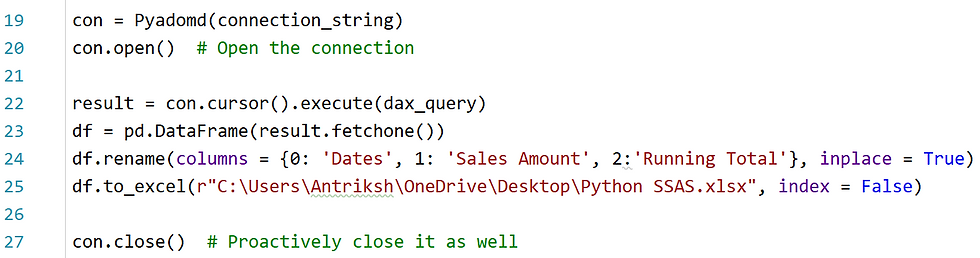top of page
Search
• Antriksh Sharma

# Using Python to Execute DAX Queries in Power BI & SSAS Tabular - Part 1

Updated: Oct 5, 2022

In this blog let's take a look at how you can use Python to connect to either Power BI or SQL Server Analysis Services to run DAX Queries.

First you need a Python IDE so that you can run the code, and then we need to import Pandas and path from sys and first let's check what all is included in path.Now we need to import Pyadomd, but if you try to do that it gives you an error that "Make sure that the dll is added, to the path, before you import Pyadomd."So first we need to add a certain dll to the path and that is '\\Program Files\\Microsoft.NET\\ADOMD.NET\\150'

I have used "=" to separate the printed code.And now you can see that, that dll has been added to the path:If during installation of pyadomd you face any issues then just run

pip install --pre pythonnet

now we need to create 2 variables that will hold the name of the data model in Power BI/SSAS and the port number/server name on which SSAS is running.

To identify the model name and port number for Power BI we can use DAX Studio, and we need to run a DMV to get the database ID.This is how the code looks like so far:Now we need to write the connection string, ther are 2 ways

either you use this one: f'Provider=MSOLAP;Data Source={port_number};Catalog={model_name};'

Or you rely on automatically generated one, which you can get from Excel.

To get the complete connection string from Excel you need to know the port number shown above and follow the steps shown below.Go to Queries & Connection and copy the connection string and modify the Source and Initial Catalog arguments in Python code.After this we need to write our DAX Query that will be executed against the data model. For the first example we can use basic query such as EVALUATE Products.Next we need to open the connection to the Power BI model:next we are going to fetch the records and load it into a data frameThis is what we get:We can run more complex quries as well that gets the running total:And now we can rename the columns and load the dataframe to Excel and if you get an error just make sure that openpyxl is installed.Succesfully exported the data:## Connecting to SSAS Tabular

Connecting to SSAS Tabular is even easier as you only need to specify the name of the data model and the server name:Now we can declare measures on the fly and run more complex queries:Once you paste the above DAX Query into the dax_query variable you will get your desired result:Complete code:

```import pandas as pd
from sys import path

model_name = 'Contoso 2022'
port_number = r'summer\antriksh'

connection_string = f'Provider=MSOLAP;Data Source={port_number};Catalog={model_name};'

dax_query = """
DEFINE
MEASURE Sales[Total Sales] =
SUMX ( Sales, Sales[Quantity] * Sales[Net Price] )

MEASURE Sales[Running Total] =
CALCULATE ( [Total Sales], DATESYTD ( Dates[Date] ) )

EVALUATE
SUMMARIZECOLUMNS (
Dates[Date],
"Sales", [Total Sales],
"Running Total", [Running Total]
)
"""
con.open()  # Open the connection

result = con.cursor().execute(dax_query)

df = pd.DataFrame(result.fetchone())

df.rename(columns = {0: 'Dates', 1: 'Sales Amount', 2:'Running Total'}, inplace = True)

df.to_excel(r"C:\Users\Antriksh\OneDrive\Desktop\Python SSAS.xlsx", index = False)

print(df)

con.close()  # Proactively close it as well```

One way of renaming the columns is to get the column names from the recordset itself and for that you can use this code:

```con = Pyadomd(connection_string) con.open()  # Open the connection
result = con.cursor().execute(dax_query)
col_names = [i.name for i in result.description]
df = pd.DataFrame(result.fetchone(), columns=col_names)```

7,249 views1 comment
bottom of page